Investigating Volume 2:

# How can centimeter cubes help us measure volume?

## Plan Investigating Volume 2

In the last session, students had a fairly easy time putting objects in volume order because the objects were dissimilar in size. In this session, the exercise becomes more difficult.

### Formative Assessment

Can students use centimeter cubes to measure the volumes of 4 blocks?

Available online at inquiryproject.terc.edu

Challenged to order four wood blocks of different shape but similar volume, students struggle to “guesstimate.” As it becomes clear that they need a standard unit of measure, students are given centimeter cubes to work with. By constructing replicas of the blocks with the cubes, they are able to check their estimates and put the blocks in proper volume order.

By the end of the investigation, students will have a firsthand appreciation of the need for a standard measure of volume, as well as a hands-on understanding of the unit “cubic centimeter.”

### Learning Goals

• to appreciate the need for a standard measure for volume
• to describe volume in cubic centimeters
Sequence of experiences
1. Think about measuring volume All Class 10 Mins
2. Place four blocks in a volume line Small Groups 15 Mins
3. Explore cubic centimeters Small Groups 5 Mins
4. Measure the volume of the blocks Small Groups 15 Mins

### Materials and Preparation

For the class:
• Post the investigation question in a place where all students can see it.
• Prepare and post a class table labeled “How We Measure Things”; an example is given in Step 1.
• Prepare and post a class table labeled “Order of Blocks by Volume”; an example is given in Step 2.
• The class volume line from the previous session (with the Styrofoam, maple block, cubes, et al.)
• Label sets of 4 wooden blocks A, B, C, D; see image in Step 1.
For each group:
• 1 set of 4 wood blocks labeled A, B, C, and D, or enough blocks for each student to have 1 block
• At least 75 cubic centimeter blocks

### Notebook Pages

Formative Assessment

## Can students use centimeter cubes to measure the volumes of 4 blocks?

Look for evidence that they can distinguish volume from other measurements as students use centimeter cubes to find the volumes of 4 blocks. Third grade students often confuse volume with area or height.

Keep these questions in mind as you interpret students' strategies:

• Do students take all 3 dimensions into account as they estimate the order of the blocks by volume?
• When they check their estimates, do they line up the cubes along one edge? Surround the block? Measure the height? Build a 3–D copy or replica?

To provide more experience with a difficult concept — volume is hard — a next step might be to pair students and ask them to explain their measurement strategies to each other.

## 1. Think about measuring volume

All Class 10 Mins

Hang on to the investigation question:  Do not refer to the investigation question until students have been introduced to the term cubic centimeter in Step 3; for now, stick with “How can we measure volume?”

Recall the work of the last session, when students thought about how much space objects take up. Draw attention to the volume line they created and pose a question:

• How could we actually measure the amount of space these objects take up?
• How can we measure their volume?

Have students brainstorm some answers. They may suggest measuring the height, or the weight, or filling the objects with water and then measuring the water in a measuring cup. Some of their ideas will be on the right track; others will not. As they grapple with the question, listen to what they say. Are they confusing weight and volume? Are they thinking three-dimensionally?

How We Measure Things
Height Centimeters
Inches
Weight Grams
Pounds
How much space (VOLUME) ???

Begin to construct the table called “How We Measure Things.”

• When we want to measure the height of something, what units do we use?
• What about weight? Distance? Temperature?

Then fill in the last line of the table: “How much space (VOLUME),” leaving question marks in the units column. Let students know that by the end of the class, they will have an answer. But first they have a new challenge.

Volume formulas:  Some students may know a formula for calculating volume. Acknowledge any algorithms that are offered, but let everyone know that the class will use a different method for measuring volume.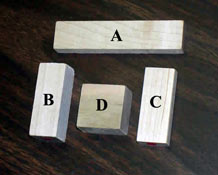Show students the four wooden blocks, and tell them their task is to put them in volume order. Emphasize that they must work together, that they will have to investigate the blocks very carefully, and that they must come to agreement before recording the order in their notebooks.

## 2. Place four blocks in a volume line

Small Groups 15 Mins Notebook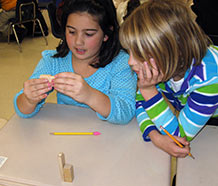Explore and Estimate: Give students about 5 minutes to explore the blocks and to range them on a volume line from “Least volume” to “Most volume.” They will quickly discover that this exercise is harder than the last one, for the volumes of the blocks are very similar.

As you circulate among the groups, ask how they are making their decisions and encourage students to think about how they might actually measure the volumes. As each group comes to consensus, have a volunteer record the order in the class table. Students should also record their order in their notebooks.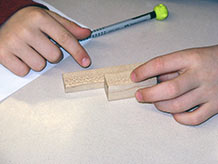When the table is complete, ask the class to study it. Do they see any patterns? Does everyone agree which cube has the greatest volume? The least volume? Did the groups use different strategies? Where are there differences of opinion? Acknowledge that the task is hard, and let students know that it’s OK to disagree because these are “guesstimates,” not measurements.

Similar volumes:  The volumes of three of the blocks are intentionally similar (or the same). As in Investigating Weight Investigation 1 (when the students tried to distinguish similar weights using only their senses), the point is to establish the value of having a measuring system that is precise and controlled.

• Does anyone have any more ideas about how we might measure volume?
Model of table for recording the order of blocks by volume
Least volume Next Next Most volume
Group 1 Order
Group 2 Order
Group 3 Order
Group 4 Order
Group 5 Order
Measured Order*

* The data for “Measured Order” will be filled in during Step 4.

The volume order of the cubes from least to most is C, A, D, B or C, D, A, B. The volume of Block C is 10 cubic centimeters. The volume of Blocks A and D is 18 cubic centimeters. The volume of Block B is 20 cubic centimeters.

## 3. Explore cubic centimeters

Small Groups 5 Mins

While students are still in their small groups, distribute enough centimeter cubes so that each student can hold several.

• Do you think we could use these tiny cubes to help us measure volume?
• How could they help use figure out the correct order of the block?

Let the students brainstorm some ideas, then introduce the unit of measure. Holding up a cube:

• This is a small cube; in fact, every edge of this cube is just 1 centimeter long. The cube takes up only a small amount of space. That amount of space is called 1 cubic centimeter, and that’s the unit of measurement that scientists use to measure volume.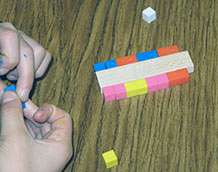Write “cubic centimeters” in the empty cell of the “How We Measure Things” table. Explain that two of these cubes take up 2 cubic centimeters of space (or “have a volume of 2 cubic centimeters”) and five cubes have a volume of 5 cubic centimeters, and so on.

• Can you think of anything that takes up about 1 cubic centimeter of space?
• Where would one of these cubes go on our volume line?

Add a cube to the line, then draw attention to the investigation question:

“How can centimeter cubes help us measure volume?”

Centimeter cubes and cubic centimeters:  Students may be confused at first about the difference between centimeter cubes and cubic centimeters. Centimeter cubes are the physical cubes, often made from wood or plastic; they have a dimension of one centimeter along each edge. A cubic centimeter is the amount of space that a centimeter cube takes up; it is a standard measure for volume.

If you sense confusion, ask, “Are you talking about the object, or are you talking about the volume (or, the amount of space it takes up or, the unit of measure)?”

## 4. Measure the volume of the blocks

Small Groups 15 Mins Notebook

Distribute the sets of centimeter cubes to each group. Have the students work together to find a way to use the cubes to determine the correct volume order of the wooden blocks.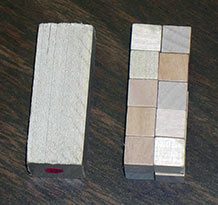By building a replica of Block Busing centimeter cubes, students can see that the volume of the block is 20 cubic centimeters.

There may be stops and starts as students reveal their misconceptions about how to measure volume. For example, they may try to measure length and width, or they may surround the perimeter of a block with the centimeter cubes.

Measure: The most successful strategy is to use the cubes to replicate a block, and then count the cubes to determine the volume. If one group discovers this strategy, they can share it with the class. Make sure each student in a group measures the volume of at least one block, using the replication strategy. Then have the group establish the volume order of the blocks based on these measurements.

After everyone has recorded the measured order their notebook, ask one student to enter the “Measured Order” in the bottom row of the class table. Make sure the entry includes the unit of measure: cubic centimeters.

Does everyone agree on the measured volumes? How do the measurements compare to the “guesstimates”? Do students see how the opportunity to measure volume allows them to order the blocks even when the differences are very small?

Finally, ask students to add one set of the blocks to the volume order line.